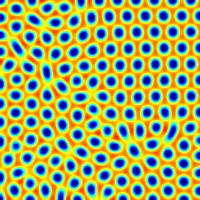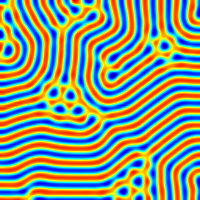# Coupled reaction–diffusion equations

The Brusselator is a theoretical model for a type of autocatalytic reaction. The Brusselator model was proposed by Ilya Prigogine and his collaborators at the Free University of Brussels.

Two chemical compounds with concentrations C_1 and C_2 interact according to the coupled reaction–diffusion equations: \displaystyle \partial_t C_1 = \nabla^2 C_1 + k(ka - (kb + 1)C_1 + C_1^2 C_2) \displaystyle \partial_t C_2 = D \nabla^2 C_2 + k(kb C_1 - C_1^2 C_2)

We will use a Cartesian (multi)grid, the generic time loop and the time-implicit diffusion solver.

#include "grid/multigrid.h"
#include "run.h"
#include "diffusion.h"

We need scalar fields for the concentrations.

scalar C1[], C2[];

We use the same parameters as Pena and Perez-Garcia, 2001

double k = 1., ka = 4.5, D = 8.;
double mu, kb;

The generic time loop needs a timestep. We will store the statistics on the diffusion solvers in mgd1 and mgd2.

double dt;
mgstats mgd1, mgd2;

## Parameters

We change the size of the domain L0 and set the tolerance of the implicit diffusion solver.

int main()
{
init_grid (128);
size (64);
TOLERANCE = 1e-4;

Here \mu is the control parameter. For \mu > 0 the system is supercritical (Hopf bifurcation). We test several values of \mu.

  mu = 0.04; run();
mu = 0.1;  run();
mu = 0.98; run();
}

## Initial conditions

event init (i = 0)
{

The marginal stability is obtained for kb = kbcrit.

  double nu = sqrt(1./D);
double kbcrit = sq(1. + ka*nu);
kb = kbcrit*(1. + mu);

The (unstable) stationary solution is C_1 = ka and C_2 = kb/ka. It is perturbed by a random noise in [-0.01:0.01].

  foreach() {
C1[] = ka ;
C2[] = kb/ka + 0.01*noise();
}
}

## Outputs

Here we create an mpeg animation of the C_1 concentration. The spread parameter sets the color scale to \pm twice the standard deviation.

event movie (i = 1; i += 10)
{
output_ppm (C1, linear = true, spread = 2, file = "f.mp4", n = 200);
fprintf (stderr, "%d %g %g %d %d\n", i, t, dt, mgd1.i, mgd2.i);
}

We make a PNG image of the final “pseudo-stationary” solution.

event final (t = 3000)
{
char name;
sprintf (name, "mu-%g.png", mu);
output_ppm (C1, file = name, n = 200, linear = true, spread = 2);
}

## Time integration

event integration (i++)
{

We first set the timestep according to the timing of upcoming events. We choose a maximum timestep of 1 which ensures the stability of the reactive terms for this example.

  dt = dtnext (1.);

We can rewrite the evolution equations as \displaystyle \partial_t C_1 = \nabla^2 C_1 + k k_a + k (C_1 C_2 - k_b - 1) C_1 \displaystyle \partial_t C_2 = D \nabla^2 C_2 + k k_b C_1 - k C_1^2 C_2 And use the diffusion solver to advance the system from t to t+dt.

  scalar r[], beta[];

foreach() {
r[] = k*ka;
beta[] = k*(C1[]*C2[] - kb - 1.);
}
mgd1 = diffusion (C1, dt, r = r, beta = beta);
foreach() {
r[] = k*kb*C1[];
beta[] = - k*sq(C1[]);
}
const face vector c[] = {D, D};
mgd2 = diffusion (C2, dt, c, r, beta);
}

## Results

We get the following stable Turing patterns.\mu=0.04 \mu=0.1 (stripes) \mu=0.98 (hexagons)

Animation of the transitions